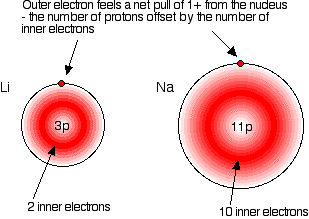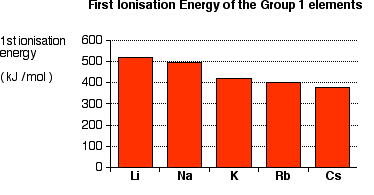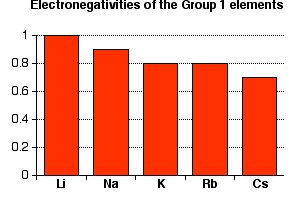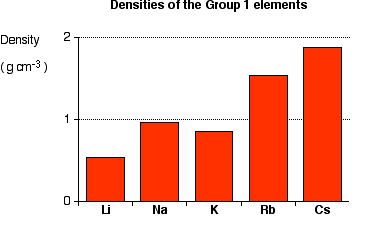# Group 1: Properties of Alkali Metals

•• Contributed by Jim Clark
• Former Head of Chemistry and Head of Science at Truro School in Cornwall

This page discusses the trends in some atomic and physical properties of the Group 1 elements - lithium, sodium, potassium, rubidium and cesium. Sections below cover the trends in atomic radius, first ionization energy, electronegativity, melting and boiling points, and density.

## Trends in Atomic Radius

The chart below shows the increase in atomic radius down the group.The radius of an atom is governed by two factors:

1. The number of layers of electrons around the nucleus
2. The attraction the outer electrons feel from the nucleus

Compare the electronic configurations of lithium and sodium:

• Li: 1s22s1
• Na: 1s22s22p63s1

In each element, the outer electron experiences a net charge of +1 from the nucleus. The positive charge on the nucleus is canceled out by the negative charges of the inner electrons. This effect is illustrated in the figure below:This is true for each of the other atoms in Group 1. The only factor affecting the size of the atom is the number of layers of inner electrons which surround the atom. More layers of electrons take up more space, due to electron-electron repulsion. Therefore, the atoms increase in size down the group.

The first ionization energy of an atom is defined as the energy required to remove the most loosely held electron from each of one mole of gaseous atoms, producing one mole of singly charged gaseous ions; in other words, it is the energy required for 1 mole of this process:

$X(g) \rightarrow X^+ (g) + e^-$A graph showing the first ionization energies of the Group 1 atoms is shown above. Notice that first ionization energy decreases down the group. Ionization energy is governed by three factors:

• the charge on the nucleus,
• the amount of screening by the inner electrons,
• the distance between the outer electrons and the nucleus.

Down the group, the increase in nuclear charge is exactly offset by the increase in the number of inner electrons. As mentioned before, in each of the elements Group 1, the outermost electrons experience a net charge of +1 from the center. However, the distance between the nucleus and the outer electrons increases down the group; electrons become easier to remove, and the ionization energy falls.

## Trends in Electronegativity

Electronegativity is a measure of the tendency of an atom to attract a bonding pair of electrons. It is usually measured on the Pauling scale, on which the most electronegative element (fluorine) is given an electronegativity of 4.0 (Table A2).A graph showing the electronegativities of the Group 1 elements is shown above. Each of these elements has a very low electronegativity when compared with fluorine, and the electronegativities decrease from lithium to cesium.

Picture a bond between a sodium atom and a chlorine atom. The bond can be considered covalent, composed of a pair of shared electrons. The electron pair will be pulled toward the chlorine atom because the chlorine nucleus contains many more protons than the sodium nucleus. This is illustrated in the figure below:The electron pair is so close to the chlorine that an effective electron transfer from the sodium atom to the chlorine atom occurs—the atoms are ionized. This strong attraction from the chlorine nucleus explains why chlorine is much more electronegative than sodium.

Now compare this with a lithium-chlorine bond. The net pull from each end of the bond is the same as before, but the lithium atom is smaller than the sodium atom. That means that the electron pair is going to be more strongly attracted to the net +1 charge on the lithium end, and thus closer to it.In some lithium compounds there is often a degree of covalent bonding that is not present in the rest of the group. Lithium iodide, for example, will dissolve in organic solvents; this is a typical property of covalent compounds. The iodine atom is so large that the pull from the iodine nucleus on the pair of electrons is relatively weak, and a fully-ionic bond is not formed.

## Summarizing the trend down the group

As the metal atoms increase in size, any bonding electron pair becomes farther from the metal nucleus, and so is less strongly attracted towards it. This corresponds with a decrease in electronegativity down Group 1. With the exception of some lithium compounds, the Group 1 elements each form compounds that can be considered ionic. Each is so weakly electronegative that in a Group 1-halogen bond, we assume that the electron pair on a more electronegative atom is pulled so close to that atom that ions are formed.

## Trends in melting and boiling pointsThe figure above shows melting and boiling points of the Group 1 elements. Both the melting and boiling points decrease down the group.

When any of the Group 1 metals is melted, the metallic bond is weakened enough for the atoms to move more freely, and is broken completely when the boiling point is reached. The decrease in melting and boiling points reflects the decrease in the strength of each metallic bond.

The atoms in a metal are held together by the attraction of the nuclei to electrons which are delocalized over the whole metal mass. As the atoms increase in size, the distance between the nuclei and these delocalized electrons increases; therefore, attractions fall. The atoms are more easily pulled apart to form a liquid, and then a gas. As previously discussed, each atom exhibits a net pull from the nuclei of +1. The increased charge on the nucleus down the group is offset by additional levels of screening electrons. As before, the trend is determined by the distance between the nucleus and the bonding electrons.

## Trends in Density

The densities of the Group 1 elements increase down the group (except for a downward fluctuation at potassium). This trend is shown in the figure below:The metals in this series are relatively light—​lithium, sodium, and potassium are less dense than water (less than 1 g cm-3). It is difficult to develop a simple explanation for this trend because density depends on two factors, both of which change down the group. The atoms are packed in the same way, so the two factors considered are how many atoms can be packed in a given volume, and the mass of the individual atoms. The amount packed depends on the individual atoms' volumes; these volumes, in turn, depends on their atomic radius.

Atomic radius increases down a group, so the volume of the atoms also increases. Fewer sodium atoms than lithium atoms, therefore, can be packed into a given volume. However, as the atoms become larger, their masses increase. A given number of sodium atoms will weigh more than the same number of lithium atoms. Therefore, 1 cm3 of sodium contains fewer atoms than the same volume of lithium, but each atom weighs more. Mathematical calculations are required to determine the densities.

## Contributors

Jim Clark (Chemguide.co.uk)